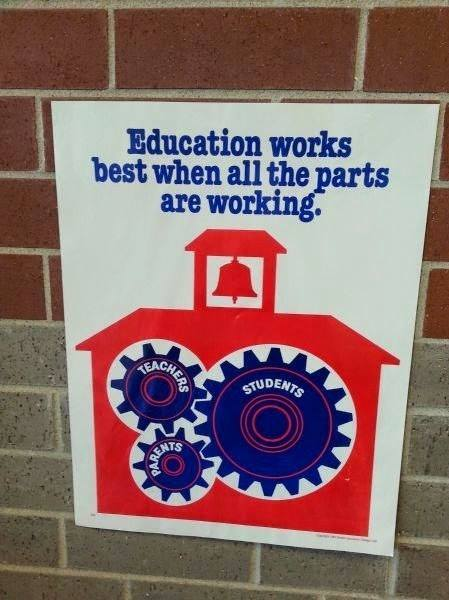# Internet Problem Solving Contest

## Solution to Problem T – Turning gears

This problem was inspired by the following “motivational” poster:The poster actually illustrates a second reason why it might be the case that gear n does not rotate. In some cases, a cluster of gears may become jammed.

But before we analyze that, let’s make a quick observation about the rotation speed of gears. The problem statement tells us how the speeds of two joined wheels are related to each other. The formula is pretty straightforward: the longer the radius (or, equivalently, the circumference) of a wheel, the slower it rotates.

We can easily visualize why the formula works as described. Imagine two linked gears: gear A with radius 1 and gear B with radius 3. Gear A is covered in fresh red paint, gear B is all white. As you rotate gear A, a longer and longer part of gear B will become red. By the time gear A makes a full revolution, what part of B’s circumference will be red? This is easy: the circumference of B is three times the circumference of A, thus exactly one third of B’s circumference will be red. And that, in turn, means that B’s speed will be one third of A’s speed.

The key to an easy solution is to realize that this also applies if there are more than two gears. Suppose you have a row of gears, each linked to the next one. If you rotate the first gear by some amount, the circumference of each gear will rotate by the same amount. This means that the observation “ratio of speeds is the inverse ratio of radii” holds for any two gears in the machine, not just adjacent gears.

In other words, if we want to find the rotation speed of the last gear, all that matters are the radii of the first and the last gear. The sizes of the remaining gears in the row do not matter at all.

This makes the solution to the easy subproblem trivial. We are guaranteed that the gears are all linked and they cannot be jammed, so gear n will definitely rotate. Its rotation speed is r1/rn rotations per second. (Remember to reduce this fraction before printing it!)

All that remains is to find its direction of rotation – in other words, to find whether its distance from gear 1 is odd or even. Any simple graph search will answer this.

For the hard subproblem, you first needed to realize that the gears can sometimes become jammed – hence phrases like “Jano will attempt to rotate gear number 1” in the problem statement.

But that was not all. You also needed to realize that a cycle in the gear network does not necessarily mean that the gears will be jammed.

For instance, take 100 identical gears and place them regularly into a 10 × 10 grid, so that each gear touches its neighbors. This grid of gears will happily rotate: half of them in one direction, half of them in the other.

In fact, the sizes of gears don’t even have to be the same. As we already know, when the gears rotate, their circumferences all shift by the same length. This means that any collection of gears will rotate happily… as long as the directions are correct. The source of the jam is when two different gears try to push a third one to rotate at the same speed but in the opposite direction.

This happens whenever the sequence of rotating gears reaches an odd cycle in the gear adjacency graph: a cyclic sequence of 2k + 1 gears in which each gear is linked to both its neighbors. In other words, the jam happens whenever the component that contains gear 1 is not bipartite.

This gives us an easy solution for both subproblems. Starting from gear 1, use any graph search (e.g., BFS or DFS) to find the rotation direction for each gear in its component. If, at any moment, you encounter a contradiction, output that the gears are jammed. Otherwise, you already know the rotation direction of gear n, and as we have shown above, you can compute its rotation speed from just the radii r1 and rn.